CAT  >  Test: CAT Quantitative Aptitude- 2

# Test: CAT Quantitative Aptitude- 2

Test Description

## 22 Questions MCQ Test CAT Mock Test Series | Test: CAT Quantitative Aptitude- 2

Test: CAT Quantitative Aptitude- 2 for CAT 2022 is part of CAT Mock Test Series preparation. The Test: CAT Quantitative Aptitude- 2 questions and answers have been prepared according to the CAT exam syllabus.The Test: CAT Quantitative Aptitude- 2 MCQs are made for CAT 2022 Exam. Find important definitions, questions, notes, meanings, examples, exercises, MCQs and online tests for Test: CAT Quantitative Aptitude- 2 below.
Solutions of Test: CAT Quantitative Aptitude- 2 questions in English are available as part of our CAT Mock Test Series for CAT & Test: CAT Quantitative Aptitude- 2 solutions in Hindi for CAT Mock Test Series course. Download more important topics, notes, lectures and mock test series for CAT Exam by signing up for free. Attempt Test: CAT Quantitative Aptitude- 2 | 22 questions in 40 minutes | Mock test for CAT preparation | Free important questions MCQ to study CAT Mock Test Series for CAT Exam | Download free PDF with solutions
 1 Crore+ students have signed up on EduRev. Have you?
*Answer can only contain numeric values
Test: CAT Quantitative Aptitude- 2 - Question 1

### Ramesh went to a food joint and bought some burgers and rolls for his family members. In total, he spent less than Rs. 3000. Had Ramesh spent the amount he actually spent on buying burgers to buy rolls and vice versa, he would have ended up buying 3 more items in total. What is the maximum number of burgers that Ramesh could have bought if it is known that a burger and a roll cost Rs. 80 and Rs. 60 respectively?

Detailed Solution for Test: CAT Quantitative Aptitude- 2 - Question 1

Let 'x' and 'y' be the number of burgers and rolls bought by Ramesh in the first case.
It is given that: 80x + 60y < 3000
⇒ 4x+3y < 150 ... (1)
We are given that in the 2nd case he spends Rs. 80x on purchasing rolls and Rs. 60y on burgers.
It is known that in the 2nd case Ramesh was able to buy 3 more items in the same amount of money. Hence,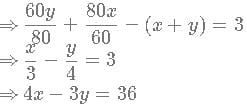At y = 4, x = 12
At y = 8, x = 15
At y = 12, x = 18
At y = 16, x = 21
At y = 20, x can't be 24 because in that case 4x+3y > 150. Hence, we can say that xmax = 21.

Test: CAT Quantitative Aptitude- 2 - Question 2

### There are two types of bread A and B; Type A bread has carbohydrates, fats and proteins and type B has carbohydrates, fats and honey. These two types of bread are mixed to form bread C. Type A and C has 30% carbohydrates. The percentage of honey in Type B and Type C is 40% and 20% respectively. Type C bread has 24% of proteins. The percentage of fats in Type A bread is

Detailed Solution for Test: CAT Quantitative Aptitude- 2 - Question 2

Type A and type C bread has 30% carbohydrates, so type B must have 30% carbohydrates.
The percentage of honey in type B and type C bread is 40% and 20%.
Let us consider 'a' grams and 'b' grams of type A and type B bread is mixed to form bread C.
40*b=20(a+b)
a=b
An equal proportion of both the types of bread is taken.
Type C bread has 24% of proteins. Since only type, A bread has proteins in it.
Let the percentage of proteins in type A be X.
X*a = 24(a+b)
X*a=24*2a
X = 48
The amount of fat in bread A = 100-30-48 = 22%

*Answer can only contain numeric values
Test: CAT Quantitative Aptitude- 2 - Question 3

### Virat, Rohit and Shikhar finished as the top 3 run scorers in IPL 2018. Virat scored half the number of runs scored by Rohit and Shikhar combined whereas Rohit scored one-fourth of what Virat and Shikar scored together. If Virat scored 150 runs more than Rohit, then the total runs scored by the 3 players is

Detailed Solution for Test: CAT Quantitative Aptitude- 2 - Question 3

Let 'V', 'R' and 'S' be the number of runs scored by Virat, Rohit and Shikar respectively.
It is given that,
⇒  V - R = 150 or V = R + 150 ... (1)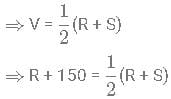⇒ 2R + 300 = R + S
⇒ S = R + 300 ... (2)
Also,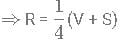⇒ 4R = R + 150 + R + 300
⇒ R = 225 Runs.
Therefore, V = R + 150 or 375 runs,   S = R + 300 = 525 runs.
Hence, the total number of runs scored by all 3 players combined = 375 + 225 + 525 = 1125.

Test: CAT Quantitative Aptitude- 2 - Question 4

Set A has 5 elements and set B has 4 elements. The total number of into functions that can be defined from set A to B is

Detailed Solution for Test: CAT Quantitative Aptitude- 2 - Question 4

A into function is one in which at-least one element in the co-domain is not connected to an element in the domain.
So, we have to exclude the possibility in which each element of co-domain gets mapped.
In other words, the difference between the total number of functions and the total number of onto functions will give us the total number of into functions.
Each element in the domain has 44 options in the co-domain. Therefore, the total number of functions that can be defined from set A to set B = 4∗4∗4∗4∗4 = 45 = 1024 = 4^54 = 1024
Total number of onto functions that can be defined from set A to B:
5 elements in the domain must be mapped to 4 elements in the co-domain.
Therefore, exactly one element in the co-domain must be mapped to 2 elements in the domain.
The element in the co-domain can be selected in 44 ways.
The 2 elements to which it gets mapped can be selected in 5C2 = 10 ways.
The remaining 3 values can be mapped in 3! = 6 ways.
Therefore, the total number of onto functions that can be defined is 4*6*10=240
Therefore, the total number of into functions = 1024 - 240 = 784.
Therefore, option D is the right answer.

*Answer can only contain numeric values
Test: CAT Quantitative Aptitude- 2 - Question 5

We are told that f(x) is a polynomial function such that f(a)f(b) = f(a) + f(b) + f(ab) - 2 and f(4) = 17, find the value of f(7).

Detailed Solution for Test: CAT Quantitative Aptitude- 2 - Question 5

f(a)f(b) = f(a) + f(b) + f(ab) - 2
Put a = b = 1.
[f(1)]2 =3f(1)−2 ⇒ f(1) = 1 (or) 2.
Let's assume f(1) = 1
Now, put b = 1.
f(a) = 2f(a) - 1
⇒ f(a) = 1 ⇒ For all values of a, f(a) = 1.
This is false because f(4) = 17.
⇒ f(1) = 2 is the correct value.
Now put b = 1/a
f(a)f(1/a) = f(a) + f(1/a) + 2 - 2
⇒ f(a)f(1/a) = f(a) + f(1/a)
So taking RHS terms to LHS and adding 1 to both sides we get
f(a)f(1/a) - f(a) - f(1/a) +1 = 1
(f(a) - 1) (f(1/a)-1) = 1
Let g(x) = f(x)-1
So g(x)*g(1/x) = 1
So g(x) is of the form ±xn
So f(x) is of the form ±xn +1.
f(a) = an +1 satisfies the above condition.
−4n +1 = 17 ⇒ -4n−4 n  = 16, which is not possible.
4a +1 = 17 ⇒ n = 2
⇒ f(a) = a2 + 1a 2 +1
⇒ f(7) = 72 + 1 = 50

*Answer can only contain numeric values
Test: CAT Quantitative Aptitude- 2 - Question 6

We are told that f(x) is a polynomial function such that f(a)f(b) = f(a) + f(b) + f(ab) - 2 and f(4) = 17, find the value of f(7).

Detailed Solution for Test: CAT Quantitative Aptitude- 2 - Question 6

f(a)f(b) = f(a) + f(b) + f(ab) - 2
Put a = b = 1.
[f(1)] 2 =3f(1)−2 ⇒ f(1) = 1 (or) 2.
Let's assume f(1) = 1
Now, put b = 1.
f(a) = 2f(a) - 1
⇒ f(a) = 1 ⇒ For all values of a, f(a) = 1.
This is false because f(4) = 17.
⇒ f(1) = 2 is the correct value.
Now put b = 1/a
f(a)f(1/a) = f(a) + f(1/a) + 2 - 2
⇒ f(a)f(1/a) = f(a) + f(1/a)
So taking RHS terms to LHS and adding 1 to both sides we get
f(a)f(1/a) - f(a) - f(1/a) +1 = 1
(f(a) - 1) (f(1/a)-1) = 1
Let g(x) = f(x)-1
So g(x)*g(1/x) = 1
So g(x) is of the form + xn
So f(x) is of the form xn + 1.
f(a) = + an + 1 satisfies the above condition.
- 4n + 1 = 17 ⇒ 4n  = 16 which is not possible.
4a +1 = 17 ⇒ n = 2
⇒ f(a) = a2 + 1
⇒ f(7) = 72 + 1 = 50

*Answer can only contain numeric values
Test: CAT Quantitative Aptitude- 2 - Question 7

A distinct number of contracts were given to each of the nine major construction companies. Find the least number of contracts that could have been given to the company that got the lowest number of contracts among the nine companies, such that sum of the number of contracts given to any five companies is greater than the sum of number of contracts given to the remaining four companies.

Detailed Solution for Test: CAT Quantitative Aptitude- 2 - Question 7

In order to minimize the total number of contracts, the number of contracts received by the companies must be consecutive integers because each of the companies got a distinct number of contracts.
So, let the number of contracts of different companies be a-4,a-3,a-2,a-1,a,a+1,a+2,a+3 and a+4.
To maintain the sum of the number of contracts given to any five companies is greater than the sum of the number of contracts given to the remaining four companies, the sum of the lowest five companies must exceed the amount of top four companies. If such a condition is maintained, then any set of 5 companies will exceed any set of 4 companies.
It is the limiting condition for five companies to exceed four companies.
5a - 10 > 4a + 10
a > 20 ⇒ Minimum value of a is 21 ⇒ Minimum value of the number of contracts got by the company which was given the least number of contracts among the 9 companies = a - 4 = 21 - 4 = 17.

Test: CAT Quantitative Aptitude- 2 - Question 8

If [X] represents the greatest integer function, a is a positive integer where [a/3]-[a/5]=2 then what is the number of possible values a can take?

Detailed Solution for Test: CAT Quantitative Aptitude- 2 - Question 8

To get the value of  [a/3]-[a/5]=2, [a/3] should be ≥2 because [a/3] ≥ [a/5]
At a=6,  [a/3]=2 but [a/5]= 1. So [a/3]-[a/5] = 1
We will take the next multiple of 3.
At a=9, [a/3]=3 but [a/5]= 1. So [a/3]-[a/5] = 2.............(1st case)
In this question, we will check the multiples of 3 and 5 as these points will change the values of [a/3] and [a/5].
Given ‘a’ is a positive integer.
We can create a following table: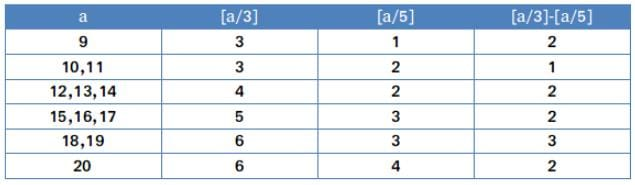For any value greater than 20 the equation [a/3]-[a/5]=2 will be greater than 3
Hence total of 8 values.

Test: CAT Quantitative Aptitude- 2 - Question 9

In a △ABC, three points P, Q and R lie on sides AB, BC and CA respectively such that AP : PB = BQ:QC = CR:RA = 3:5. Find out the ratio of the area of \triangle△PQR to that of △ABC.

Detailed Solution for Test: CAT Quantitative Aptitude- 2 - Question 9

Let us draw the diagram according to the information given in the question.
Let '8x', '8y' and '8z' be the length of side AB, BC and CA respectively.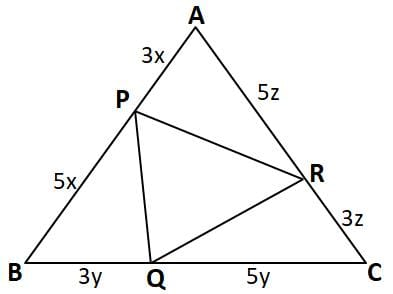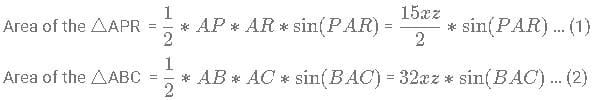From equation (1) and (2) we can say that,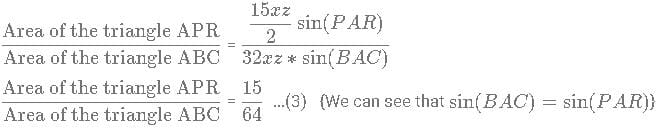Similarly,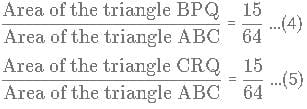We know that, Area of △PQR =  Area of the △ABC - (Area of the △APR + Area of the △BPQ + Area of the △CRQ)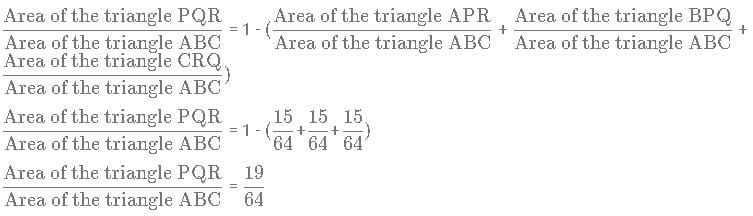Hence, option C is the correct answer.

Test: CAT Quantitative Aptitude- 2 - Question 10

In a quadilateral PQRS, T is a point on the side PQ such that \angle∠PST = \angle∠STR = \angle∠TRQ = 45°. If PS = 16 cm and RQ = 24 cm then find out the area of triangle TRS?

Detailed Solution for Test: CAT Quantitative Aptitude- 2 - Question 10

We are given that ∠PST = ∠STR ,therefore, we can say that PS ║ TR
Similarly, ∠STR = ∠TRQ, TS ║QR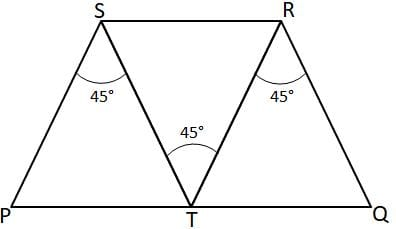In triangles PST and TRQ,
TS ║QR PS ║TR and ∠PST = ∠TRQ
Hence, we can say that △PST ∼ △TRQ.
Therefore, we can say that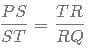⇒ ST*TR = PS*RQ  = 16*24 = 384 sq. cm
We know that area of triangle TRS =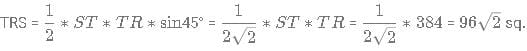cm
Thus, option D is the correct answer.

Test: CAT Quantitative Aptitude- 2 - Question 11

A wire which was used to form a square of side 30 cm is divided into 3 parts such that the length of the longest part is 25% more than length of the second longest part. If the shortest part is 20 cm shorter than the longest part, then the area (in cm2) of the triangle formed by these three parts is

Detailed Solution for Test: CAT Quantitative Aptitude- 2 - Question 11

The length of the wire = 4*30 = 120 cm.
Let 'x' be the length of the smallest part of the wire.
Length of the longest part = x + 20
Length of the second longest part*1.25 = Length of the longest part
⇒ Length of the second longest part = 0.8*Length of the longest part
⇒ x + 20 + 0.8*(x+20) + x = 120
⇒ x + 20 + 0.8x + 16 + x = 120
2.8x + 36 = 120
2.8x = 84
⇒ x = 30 cm
The sides of the triangle are 30 cm, 40 cm, and 50 cm.
We can see that the sides of the triangle form a Pythagorean triplet.
Therefore, the area of the triangle is 0.5*30*40 = 600 cm2
Therefore, option D is the right answer.

Test: CAT Quantitative Aptitude- 2 - Question 12

When the number of sides of a polygon increases from 'n' to 'n+1', A is the change in the sum of exterior angles and B is the change in the sum of interior angles. Which of the following statements is true?

Detailed Solution for Test: CAT Quantitative Aptitude- 2 - Question 12

The sum of the exterior angles of any polygon is 360 degrees. So, there will be no change in the sum of the exterior angles. The sum of the interior angles of an 'n' sided polygon is (n-2)*180. Hence, if the number of sides is increased by 1, the sum of the interior angles increases by 180.
Therefore, A = 0 and B = 180.

Test: CAT Quantitative Aptitude- 2 - Question 13

For any point, in the first quadrant, on the line 4x + 9y = 36, what is the maximum value of x6 * y6 ?

Detailed Solution for Test: CAT Quantitative Aptitude- 2 - Question 13

4x + 9y = 36
Both x and y are positive in the first quadrant ⇒ AM ≥ GM inequality holds true.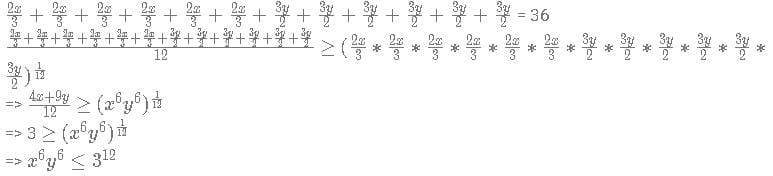Test: CAT Quantitative Aptitude- 2 - Question 14

Find the area of the region enclosed between the lines y=2|x|+3 and y=-2|x|+8.

Detailed Solution for Test: CAT Quantitative Aptitude- 2 - Question 14

First plot the two lines on a graph.
Now, find the point of intersection of y=2x+3 and y=-2x+8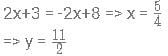So, the lines intersect at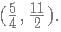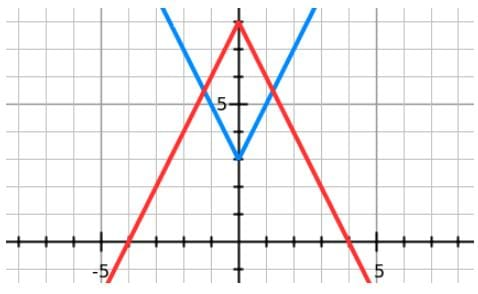If you observe carefully, the figure enclosed in by the lines is a rhombus.
The area of a rhombus is equal to half the product of its diagonals.
The length of the first diagonal is equal to 8 - 3 = 5 units.
The length of the second diagonal is equal to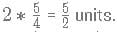Hence, the area of the enclosed figure is equal to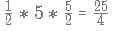square units.

Test: CAT Quantitative Aptitude- 2 - Question 15

If it is known that xx and yy are two positive numbers such that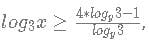what is the minimum value of x + y ?

Detailed Solution for Test: CAT Quantitative Aptitude- 2 - Question 15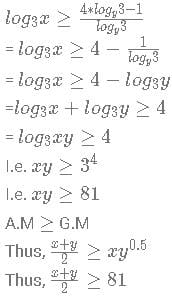Hence, x+y ≥ 18
Hence, option C is the correct answer.

Test: CAT Quantitative Aptitude- 2 - Question 16

If   log m=300\times×log1.0033333..
What is the approximate value of m?

Detailed Solution for Test: CAT Quantitative Aptitude- 2 - Question 16

log m = 300 log(1.003333)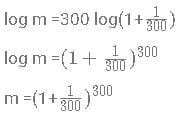Binomial Expansion of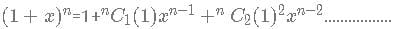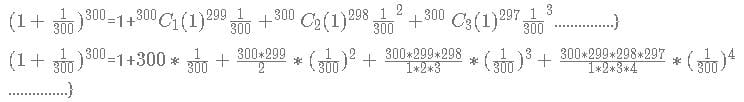=1+1+0.498+0.165+ 0.041....
(Since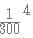and the succeeding terms are very small, they can be ignored because the fraction at the denominator is increasing and at the numerator is decreasing with the subsequent terms of bionomial.
Further if we observe the fourth term of the binomial expansion: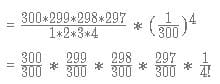we can observe that factors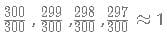and with every subsequent term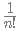will get smaller. Thus we can neglect those terms of the expansion. )
=1+1+0.498+0.165+ 0.041....
= {2.704+....}
This can be approximated to 2.7
Hence, C is the correct answer.
In general, when nn is very large, the value of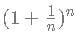tends to the constant 'e' which is approximately equal to 2.718

*Answer can only contain numeric values
Test: CAT Quantitative Aptitude- 2 - Question 17

Three positive numbers a, b and c are such that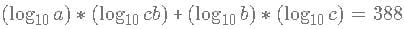and abc = 1099. Find the value of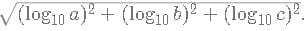Detailed Solution for Test: CAT Quantitative Aptitude- 2 - Question 17

We are given that abc = 1099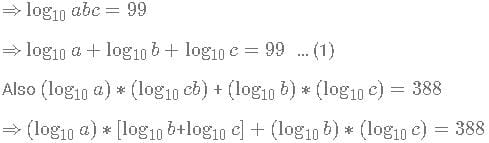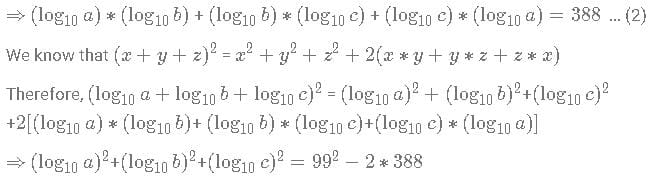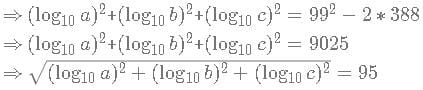*Answer can only contain numeric values
Test: CAT Quantitative Aptitude- 2 - Question 18

N is a multiple of 72. Also, it is known that all the digits of N are different. What is the largest possible value of N?

Detailed Solution for Test: CAT Quantitative Aptitude- 2 - Question 18

We know that the number is a multiple of 72. Therefore, the number must be a multiple of both 8 and 9.
If the number contains all the digits from 0 to 9, the sum of the digits will be 9*10/2 = 45.
Therefore, a number containing all the ten digits will be divisible by 9.
For a number to be divisible by 8, the last 3 digits should be divisible by 8.
Now, we have to find the largest possible number. Therefore, the left-most digit must be 9, the second digit from the left should be 8 and so on.
We'll get 9876543210 as the number. However, the last 3 digits are not divisible by 8. We must try to make the last 3 digits divisible by 8 without altering the position of other numbers. '120' is divisible by 8.
Therefore, the largest number with different digits divisible by 72 is 9876543120.

Test: CAT Quantitative Aptitude- 2 - Question 19

The number of ways in which 33 identical pens can be distributed among three boys such that each of them receives an odd number of pens is

Detailed Solution for Test: CAT Quantitative Aptitude- 2 - Question 19

Let 2a+1, 2b+1, 2c+1 be the number of pens received by each of the three boys.
2a+1+2b+1+2c+1=33  [0 ≤ a, b, c ≤ 15]
2a+2b+2c=30
a+b+c=15
Required number of ways  = 15 + 3 - 1C3 - 1
= 17C2
= 136

*Answer can only contain numeric values
Test: CAT Quantitative Aptitude- 2 - Question 20

A shopkeeper has 5 dozens of apples. On selling 12 apples for Rs.600, he incurs a loss equal to the cost price of 2 apples. At what total price should he mark the remaining 4 dozens so that even after giving 20% discount, he would have an overall gain of 10%?

Detailed Solution for Test: CAT Quantitative Aptitude- 2 - Question 20

CP = SP + loss
So, CP of 12 apples = SP of 12 apples+ CP of 2 apples
⇒ CP of 10 apples = SP of 12 apples
SP of 12 apples = Rs.600
⇒ CP of 10 apples = Rs.600
CP of 1 apple = Rs. 60
Total CP = Rs. (5 * 12 * 60) = Rs. 3600
Required overall gain = 10%
Required total SP = Rs. 3600 + 10% of Rs. 3600 = Rs. 3960
Remaining apples = 4 dozens
Required SP for 4 dozens = Rs. (3960 - 600) = Rs.3360
According to the question,
MP - 20% of MP = Rs. 3360
Or, MP = Rs. 4200
Hence, 4200 is the correct answer.

*Answer can only contain numeric values
Test: CAT Quantitative Aptitude- 2 - Question 21

An investor is faced with a dilemma about where to invest his savings of 10 lakhs. Scheme A gives 10% interest compounded annually for 2 years and simple interest of 20% for the next 3 years. If the amount has increased less than 60%, additional 10% is also added to it. Scheme B gives a simple interest of 10% for 2 years and compound interest of 20% for 3 years compounded annually. If the amount has increased less than 50%, additional 15% is added to it. If the investor goes with the right choice, how much does he earn over his initial investment ?

Detailed Solution for Test: CAT Quantitative Aptitude- 2 - Question 21

To make the calculation easier, let us take the amount the investor wants to invest as x.
Scheme A :
Amount of the investment after 2 years, a2 = x * (1.1)2 = 1.21x
Amount of investment after 5 years, a5 = 1.21x * (1 + (0.2 x 3)) = 1.936x
Since the increase is more than 60%, the additional 10% won’t be added to it.
Scheme B :
Amount of investment after 2 years, b2 = x * (1 + (0.1 x 2)) = 1.2x
Amount of investment after 5 years, b5 = 1.2x (1.2)3 = 2.0736x
Since the increase is more than 50%, the additional 15% won’t be added to it.
After looking at the interest earned, we can say that the investor should choose scheme B.
Amount earned by the investor = 106
×(2.0736−1)=1073600

*Answer can only contain numeric values
Test: CAT Quantitative Aptitude- 2 - Question 22

An infinite geometric progression has the sum 10. Sum of all the possible integral values of the first term is

Detailed Solution for Test: CAT Quantitative Aptitude- 2 - Question 22

Let us consider the first term of GP=a
Common ratio=r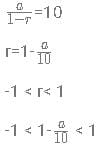0 < a < 20 but the value of a cannot be 10
Sum of possible integral values of a =Sum of first 19 Natural Numbers - 10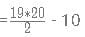=180

## CAT Mock Test Series

2 videos|24 docs|89 tests
 Use Code STAYHOME200 and get INR 200 additional OFF Use Coupon Code
Information about Test: CAT Quantitative Aptitude- 2 Page
In this test you can find the Exam questions for Test: CAT Quantitative Aptitude- 2 solved & explained in the simplest way possible. Besides giving Questions and answers for Test: CAT Quantitative Aptitude- 2, EduRev gives you an ample number of Online tests for practice

## CAT Mock Test Series

2 videos|24 docs|89 tests

### How to Prepare for CAT

Read our guide to prepare for CAT which is created by Toppers & the best Teachers